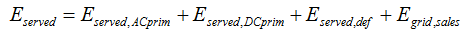# HOMER Pro 3.10

 Navigation: Glossary Total Electrical Load ServedType: Output Variable Units: kWh/yr Symbol: Eserved

The total electrical load served is the total amount of energy that went towards serving the primary and deferrable loads during the year, plus the amount of energy sold to the grid. HOMER calculates the total electrical load served using the following equation:where: Eserved,primAC = AC primary load served [kWh/yr] Eserved,primDC = DC primary load served [kWh/yr] Eserved,def = deferrable load served [kWh/yr] Egrid,sales = energy sold to the grid [kWh/yr]

HOMER uses this value to calculate the renewable fraction and the levelized cost of energy.

See also

Renewable Fraction

Levelized Cost of Energy

Thermal Load Served